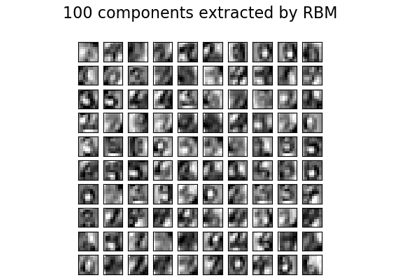/scikit-learn

# sklearn.neural_network.BernoulliRBM

`class sklearn.neural_network.BernoulliRBM(n_components=256, learning_rate=0.1, batch_size=10, n_iter=10, verbose=0, random_state=None)` [source]

Bernoulli Restricted Boltzmann Machine (RBM).

A Restricted Boltzmann Machine with binary visible units and binary hidden units. Parameters are estimated using Stochastic Maximum Likelihood (SML), also known as Persistent Contrastive Divergence (PCD) .

The time complexity of this implementation is `O(d ** 2)` assuming d ~ n_features ~ n_components.

Read more in the User Guide.

Parameters: `n_components : int, optional` Number of binary hidden units. `learning_rate : float, optional` The learning rate for weight updates. It is highly recommended to tune this hyper-parameter. Reasonable values are in the 10**[0., -3.] range. `batch_size : int, optional` Number of examples per minibatch. `n_iter : int, optional` Number of iterations/sweeps over the training dataset to perform during training. `verbose : int, optional` The verbosity level. The default, zero, means silent mode. `random_state : integer or RandomState, optional` A random number generator instance to define the state of the random permutations generator. If an integer is given, it fixes the seed. Defaults to the global numpy random number generator. `intercept_hidden_ : array-like, shape (n_components,)` Biases of the hidden units. `intercept_visible_ : array-like, shape (n_features,)` Biases of the visible units. `components_ : array-like, shape (n_components, n_features)` Weight matrix, where n_features in the number of visible units and n_components is the number of hidden units.

#### References

 Hinton, G. E., Osindero, S. and Teh, Y. A fast learning algorithm for
deep belief nets. Neural Computation 18, pp 1527-1554. http://www.cs.toronto.edu/~hinton/absps/fastnc.pdf
 Tieleman, T. Training Restricted Boltzmann Machines using
Approximations to the Likelihood Gradient. International Conference on Machine Learning (ICML) 2008

#### Examples

```>>> import numpy as np
>>> from sklearn.neural_network import BernoulliRBM
>>> X = np.array([[0, 0, 0], [0, 1, 1], [1, 0, 1], [1, 1, 1]])
>>> model = BernoulliRBM(n_components=2)
>>> model.fit(X)
BernoulliRBM(batch_size=10, learning_rate=0.1, n_components=2, n_iter=10,
random_state=None, verbose=0)
```

#### Methods

 `fit`(X[, y]) Fit the model to the data X. `fit_transform`(X[, y]) Fit to data, then transform it. `get_params`([deep]) Get parameters for this estimator. `gibbs`(v) Perform one Gibbs sampling step. `partial_fit`(X[, y]) Fit the model to the data X which should contain a partial segment of the data. `score_samples`(X) Compute the pseudo-likelihood of X. `set_params`(**params) Set the parameters of this estimator. `transform`(X) Compute the hidden layer activation probabilities, P(h=1|v=X).
`__init__(n_components=256, learning_rate=0.1, batch_size=10, n_iter=10, verbose=0, random_state=None)` [source]
`fit(X, y=None)` [source]

Fit the model to the data X.

Parameters: `X : {array-like, sparse matrix} shape (n_samples, n_features)` Training data. `self : BernoulliRBM` The fitted model.
`fit_transform(X, y=None, **fit_params)` [source]

Fit to data, then transform it.

Fits transformer to X and y with optional parameters fit_params and returns a transformed version of X.

Parameters: `X : numpy array of shape [n_samples, n_features]` Training set. `y : numpy array of shape [n_samples]` Target values. `X_new : numpy array of shape [n_samples, n_features_new]` Transformed array.
`get_params(deep=True)` [source]

Get parameters for this estimator.

Parameters: `deep : boolean, optional` If True, will return the parameters for this estimator and contained subobjects that are estimators. `params : mapping of string to any` Parameter names mapped to their values.
`gibbs(v)` [source]

Perform one Gibbs sampling step.

Parameters: `v : array-like, shape (n_samples, n_features)` Values of the visible layer to start from. `v_new : array-like, shape (n_samples, n_features)` Values of the visible layer after one Gibbs step.
`partial_fit(X, y=None)` [source]

Fit the model to the data X which should contain a partial segment of the data.

Parameters: `X : array-like, shape (n_samples, n_features)` Training data. `self : BernoulliRBM` The fitted model.
`score_samples(X)` [source]

Compute the pseudo-likelihood of X.

Parameters: `X : {array-like, sparse matrix} shape (n_samples, n_features)` Values of the visible layer. Must be all-boolean (not checked). `pseudo_likelihood : array-like, shape (n_samples,)` Value of the pseudo-likelihood (proxy for likelihood).

#### Notes

This method is not deterministic: it computes a quantity called the free energy on X, then on a randomly corrupted version of X, and returns the log of the logistic function of the difference.

`set_params(**params)` [source]

Set the parameters of this estimator.

The method works on simple estimators as well as on nested objects (such as pipelines). The latter have parameters of the form `<component>__<parameter>` so that it’s possible to update each component of a nested object.

Returns: self
`transform(X)` [source]

Compute the hidden layer activation probabilities, P(h=1|v=X).

Parameters: `X : {array-like, sparse matrix} shape (n_samples, n_features)` The data to be transformed. `h : array, shape (n_samples, n_components)` Latent representations of the data.

## Examples using `sklearn.neural_network.BernoulliRBM`Restricted Boltzmann Machine features for digit classification